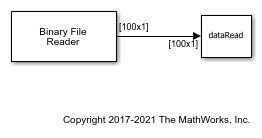# Write and Read Fixed-Point Data from Binary Files in Simulink

The Binary File Writer and Binary File Reader blocks do not support writing and reading fixed-point data. As a workaround, you can write the stored integer portion of the `fi` data, read the data, and use this value to reconstruct the `fi` data.

### Write the Fixed-Point Data

Create a `fi` object to represent 100 signed random numbers with a word length of 14 and a fraction length of 12.

```data = randn(100,1); fiDataWriter = fi(data,1,14,12); storeIntData = storedInteger(fiDataWriter); ```

Write the stored integer portion of the `fi` object to the data file `myFile.dat`. The built-in data type is `int16`, which can be computed using `class(storeIntData)`.

```writeModel = 'writeFixedData'; open_system(writeModel) sim(writeModel) ```### Read the Fixed-Point Data

Specify the reader to read the stored integer data as `int16` data with 100 samples per data frame.

```readModel = 'readFixedData'; open_system(readModel) sim(readModel) ```The real-world value of the fixed-point number can be represented using `2^[-fractionLength*storedInteger]`. If you know the signedness, word length, and fraction length of the fixed-point data, you can reconstruct the `fi` data using `fi(realValue,signedness,wordLength,fractionLength)`. In this example, the data is signed with a word length of 14 and a fraction length of 12.

```fractionLength = 12; wordLength = 14; realValue = 2^(-fractionLength)*double(dataRead); fiDataReader = fi(realValue,1,wordLength,fractionLength); ```

Verify that the writer data is same as the reader data.

```isequal(fiDataWriter,fiDataReader) ```
```ans = logical 1 ```